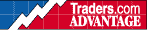HOT TOPICS LIST

INDICATORS LIST

LIST OF TOPICS

# Correlation Analysis

04/02/00 02:05:49 PM
by Jason K. Hutson

Correlation Analysis

Security:   N/A
Position:   N/A

 Correlation analysis measures the degree of relationship between two variables. The correlation coefficient r is one popular statistical index for this purpose. The values for r range between +1 to -1; a +1 reading indicates that the two variables are perfectly correlated, while a -1 reading indicates a perfect inverse relationship. An r of 0 indicates that the two variables have no correlation. The correlation coefficient r measures the relationship between two variables that are believed to be linear. For instance, a straight line is used to describe the relationship if the two variables were plotted on a graph using an X and Y axis. Sometimes, two variables will have a relationship that is described by a line that curves. The use of the formula for r isrestricted to relationships that are linear.

Jason K. Hutson

Traders.com Staff Writer. Enjoys trendlines, support and resistance, moving averages, RSI, MACD, ADX, Bollinger bands, parabolic SAR, chart formations, and volume analysis.

 Comments or Questions? Article Usefulness 5 (most useful) 4 3 2 1 (least useful)